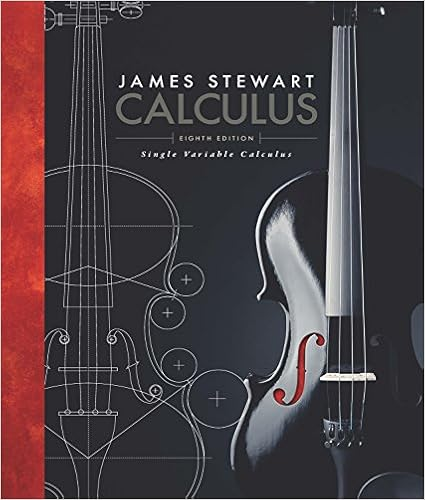# WA2(1) - Name College ID Thomas Edison State College...

• Homework Help
• 5
• 67% (6) 4 out of 6 people found this document helpful

This preview shows page 1 - 5 out of 5 pages.

##### We have textbook solutions for you!
The document you are viewing contains questions related to this textbook.The document you are viewing contains questions related to this textbook.
Chapter 7 / Exercise 22
Single Variable Calculus
StewartExpert Verified
Name:College ID:Thomas Edison State CollegeCalculus II (MAT-232)Section no.:Semester and year:Written Assignment 2Answer all assigned exercises, and show all work. Each exercise is worth 4 points.Section 6.24.Evaluate the integral.
6.Evaluate the integral.
18.Evaluate the integral.
22.Evaluate the integral.
##### We have textbook solutions for you!
The document you are viewing contains questions related to this textbook.The document you are viewing contains questions related to this textbook.
Chapter 7 / Exercise 22
Single Variable Calculus
StewartExpert Verified
24.Evaluate the integral.
46.Evaluate the integral using integration by parts and substitution.
Section 6.38.Evaluate the integral.
10.Evaluate the integral.
18.Evaluate the integral.
28.Evaluate the integral.WA 2, p. 2
30.Evaluate the integral.
Section 6.42.Find the partial fractions decomposition and an antiderivative.
12. Find the partial fractions decomposition and an antiderivative.
16. Find the partial fractions decomposition and an antiderivative.
22. Evaluate the integral.WA 2, p. 3
24. Evaluate the integral.
•••